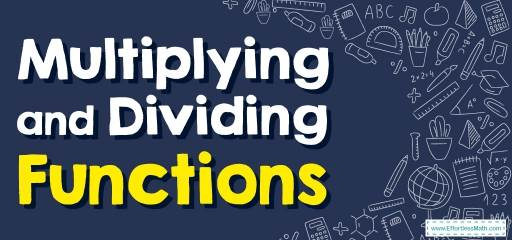# How to Multiply and Dividing Functions? (+FREE Worksheet!)

Multiplying and dividing functions are similar to multiplying and dividing polynomials. Learn how to multiply and divide functions in this post.## Step by step guide to Multiplying and Dividing Functions

• Just like we can multiply and divide numbers, we can multiply and divide functions. For example, if we had functions $$f$$ and $$g$$, we could create two new functions: $$f × g$$, and $$\frac{f}{g}$$.

Best Algebra Prep Resource

### Multiplying and Dividing Functions – Example 1:

$$g(x)=x+1, f(x)=x-2$$, Find: $$(g.f)(2)$$

Solution:

$$(g.f)(x)=g(x).f(x)=(x+1)(x-2)=x^2-2x+x-2=x^2-x-2$$
Substitute $$x$$ with $$2$$:
$$(g.f)(2)=x^2-x-2=(2)^2-2-2=4-2-2=4-4=0$$

### Multiplying and Dividing Functions – Example 2:

$$f(x)=x-2, h(x)=x+8$$, Find: $$(\frac{f}{h})(-1)$$

Solution:

$$(\frac{f}{h})(x)=\frac{f(x)}{h(x)} =\frac{x-2}{x+8}$$
Substitute $$x$$ with $$-1: (\frac{f}{h})(-1)=\frac{x-2}{x+8}=\frac{(-1)-2}{(-1)+8}=\frac{-3}{7}=-\frac{3}{7}$$

### Multiplying and Dividing Functions – Example 3:

$$g(x)=-x-2, f(x)=2x+1$$, Find: $$(g.f)$$$$(x)$$

Solution:

$$(g.f)(x)=g(x).f(x)=(-x-2)(2x+1)=-2x^2-x-4x-2=-2x^2-5x-2$$

A Perfect Practice Workbook for Algebra I

### Multiplying and Dividing Functions – Example 4:

$$f(x)=x+4, h(x)=5x-2$$, Find: $$(\frac{f}{h})$$$$(x)$$

Solution:

$$(\frac{f}{h})(x)=\frac{f(x)}{h(x)} =\frac{x+4}{5x-2}$$

## Exercises for Multiplying and Dividing Functions

### Perform the indicated operation.

• $$\color{blue}{g(a) = 2a – 1 \\ h(a) = 3a – 3 \\ Find \ (g.h)(– 4) } \\\$$
• $$\color{blue}{f(x) = 2x^3 – 5x^2 \\ g(x) = 2x – 1 \\ Find \ (f.g)(x)} \\\$$
• $$\color{blue}{g(t) = t^2 + 3 \\ h(t) = 4t – 3 \\ Find \ (g.h)(– 1)} \\\$$
• $$\color{blue}{g(n) = n^2 + 4 + 2n \\ h(n) = – 3n + 2 \\ Find \ (g.h)(1)} \\\$$
• $$\color{blue}{g(a) = 3a + 2 \\ f(a) = 2a – 4 \\ Find (\frac{g}{f})(3) } \\\$$
• $$\color{blue}{f(x) = 3x – 1 \\ g(x) = x^2 – x \\ Find \ (\frac{f}{g})(x)}$$

• $$\color{blue}{135}$$
• $$\color{blue}{4x^4 – 12x^3 + 5x^2}$$
• $$\color{blue}{-28}$$
• $$\color{blue}{-7}$$
• $$\color{blue}{\frac{11}{2}} \\\$$
• $$\color{blue}{\frac{3x-1}{x^2-x}}$$

The Absolute Best Books to Ace Algebra

### What people say about "How to Multiply and Dividing Functions? (+FREE Worksheet!) - Effortless Math: We Help Students Learn to LOVE Mathematics"?

No one replied yet.

X
45% OFF

Limited time only!

Save Over 45%

SAVE $40 It was$89.99 now it is \$49.99